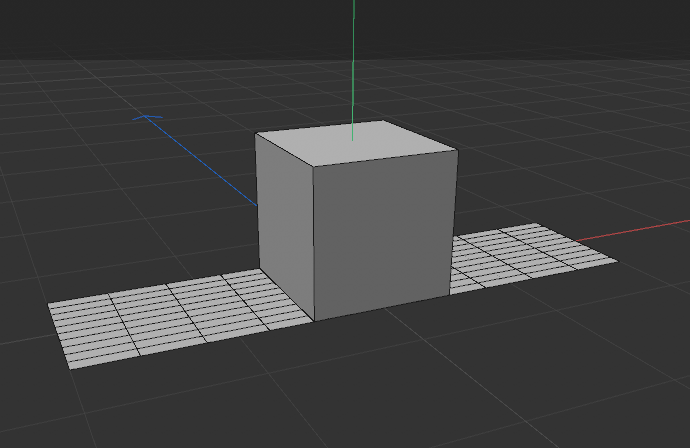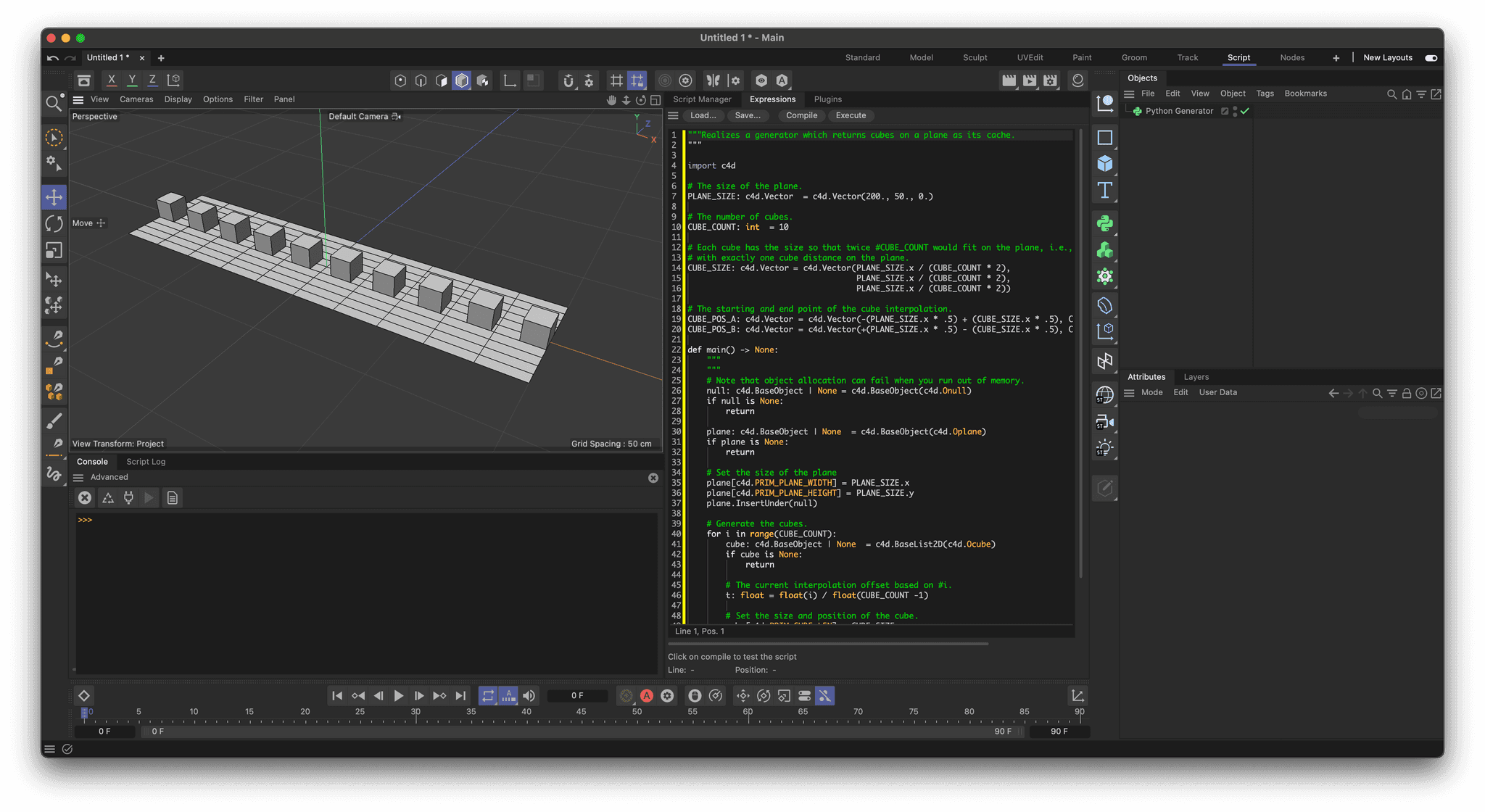# Solved Uniform distribution of objects along the length of the center of plane.

Hi all. Please tell me how can I achieve a uniform distribution of cubes along the length of another object, for example, from the center of the plane. The number of cubes is controlled by the number of iterations in the loop.

my code:

``````def main() -> None:
null = c4d.BaseObject(c4d.Onull)

#Plane
pl = c4d.BaseObject(c4d.Oplane)
plW = pl[c4d.PRIM_PLANE_WIDTH] = 200
plH = pl[c4d.PRIM_PLANE_HEIGHT] = 50
bbPl = pl.GetRad() # plane Bounding box
pl.InsertUnder(null)

#BASE-CUBE
for i in range(2):

cube = c4d.BaseList2D(c4d.Ocube)
xSize = cube[c4d.PRIM_CUBE_LEN,c4d.VECTOR_X] = 50
ySize = cube[c4d.PRIM_CUBE_LEN,c4d.VECTOR_Y] = 50
zSize = cube[c4d.PRIM_CUBE_LEN,c4d.VECTOR_Z] = 50
cube[c4d.ID_BASEOBJECT_REL_POSITION,c4d.VECTOR_Y] = ySize/2
cube[c4d.ID_BASEOBJECT_REL_POSITION,c4d.VECTOR_X] = ???
cube.InsertUnder(null)

return null
``````Hello @SmetK,

Thank you for reaching out to us. Computing a uniform distribution is a mathematical question and therefore out of scope of support, please refer to Wikipedia and other sources for mathematical concepts. The general answer is linear interpolation, which is of course just another way to say uniform distribution.

Note that the Cinema 4D API has no `lerp` function (abbreviation for linear interpolation), as one might be used to from other APIs. `c4d.utils.MixVec` provides linear interpolation for vectors and can be used as a standin.

I have provided a simple example below.

Cheers,
Ferdinand

Result:Code:

``````"""Realizes a generator which returns cubes on a plane as its cache.
"""

import c4d

# The size of the plane.
PLANE_SIZE: c4d.Vector  = c4d.Vector(200., 50., 0.)

# The number of cubes.
CUBE_COUNT: int  = 10

# Each cube has the size so that twice #CUBE_COUNT would fit on the plane, i.e., we will place cubes
# with exactly one cube distance on the plane.
CUBE_SIZE: c4d.Vector = c4d.Vector(PLANE_SIZE.x / (CUBE_COUNT * 2),
PLANE_SIZE.x / (CUBE_COUNT * 2),
PLANE_SIZE.x / (CUBE_COUNT * 2))

# The starting and end point of the cube interpolation.
CUBE_POS_A: c4d.Vector = c4d.Vector(-(PLANE_SIZE.x * .5) + (CUBE_SIZE.x * .5), CUBE_SIZE.y *.5, 0)
CUBE_POS_B: c4d.Vector = c4d.Vector(+(PLANE_SIZE.x * .5) - (CUBE_SIZE.x * .5), CUBE_SIZE.y *.5, 0)

def main() -> None:
"""
"""
# Note that object allocation can fail when you run out of memory.
null: c4d.BaseObject | None = c4d.BaseObject(c4d.Onull)
if null is None:
return

plane: c4d.BaseObject | None  = c4d.BaseObject(c4d.Oplane)
if plane is None:
return

# Set the size of the plane
plane[c4d.PRIM_PLANE_WIDTH] = PLANE_SIZE.x
plane[c4d.PRIM_PLANE_HEIGHT] = PLANE_SIZE.y
plane.InsertUnder(null)

# Generate the cubes.
for i in range(CUBE_COUNT):
cube: c4d.BaseObject | None  = c4d.BaseList2D(c4d.Ocube)
if cube is None:
return

# The current interpolation offset based on #i.
t: float = float(i) / float(CUBE_COUNT -1)

# Set the size and position of the cube.
cube[c4d.PRIM_CUBE_LEN] = CUBE_SIZE
cube[c4d.ID_BASEOBJECT_REL_POSITION] = c4d.utils.MixVec(CUBE_POS_A, CUBE_POS_B, t)

cube.InsertUnder(null)

return null
``````

MAXON SDK Specialist
developers.maxon.net

@ferdinand Thanks a lot. Very useful information. You are the best!